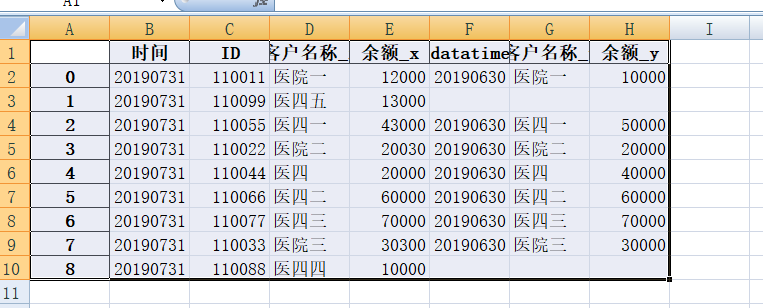python中使用merge拼接数据之后的数据处理问题？

``````import pandas as pd
table = kehus.merge(yues,how='left',on='ID')
#print(table)
table.to_excel(r'd:\test\test003.xlsx')
``````2个回答

``````from pandas import DataFrame
import pandas as pd
import numpy as np

def sumColumn(a, b):
return a + b

df = DataFrame(np.random.randn(4, 5), columns=['A', 'B', 'C', 'D', 'E'])

df['Row_sum'] = df.apply(lambda row: sumColumn(row['B'], row['C']), axis=1)
print (df)

``````